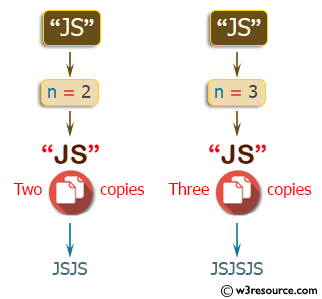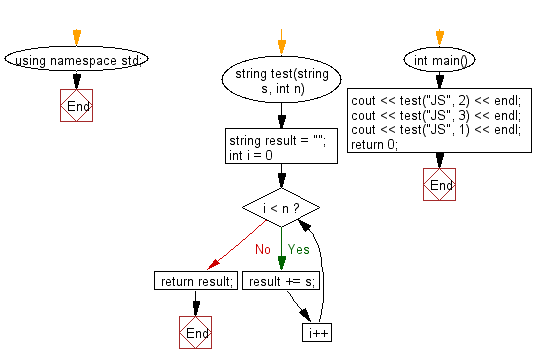﻿ C++ : Create a new string which is n copies of a string# C++ Exercises: Create a new string which is n copies of a given string

## C++ Basic Algorithm: Exercise-24 with Solution

Write a C++ program to create a new string which is n (non-negative integer) copies of a given string.

Sample Solution:

C++ Code :

``````#include <iostream>
using namespace std;

string test(string s, int n)
{
string result = "";
for (int i = 0; i < n; i++)
{
result += s;
}
return result;
}

int main()
{
cout << test("JS", 2) << endl;
cout << test("JS", 3) << endl;
cout << test("JS", 1) << endl;
return 0;
}
``````

Sample Output:

```JSJS
JSJSJS
JS
```

Pictorial Presentation:Flowchart:C++ Code Editor:

Contribute your code and comments through Disqus.

What is the difficulty level of this exercise?

﻿